Test: State Variable Analysis- 2

# Test: State Variable Analysis- 2 - Electrical Engineering (EE)

Test Description

## 10 Questions MCQ Test GATE Electrical Engineering (EE) 2024 Mock Test Series - Test: State Variable Analysis- 2

Test: State Variable Analysis- 2 for Electrical Engineering (EE) 2023 is part of GATE Electrical Engineering (EE) 2024 Mock Test Series preparation. The Test: State Variable Analysis- 2 questions and answers have been prepared according to the Electrical Engineering (EE) exam syllabus.The Test: State Variable Analysis- 2 MCQs are made for Electrical Engineering (EE) 2023 Exam. Find important definitions, questions, notes, meanings, examples, exercises, MCQs and online tests for Test: State Variable Analysis- 2 below.
Solutions of Test: State Variable Analysis- 2 questions in English are available as part of our GATE Electrical Engineering (EE) 2024 Mock Test Series for Electrical Engineering (EE) & Test: State Variable Analysis- 2 solutions in Hindi for GATE Electrical Engineering (EE) 2024 Mock Test Series course. Download more important topics, notes, lectures and mock test series for Electrical Engineering (EE) Exam by signing up for free. Attempt Test: State Variable Analysis- 2 | 10 questions in 30 minutes | Mock test for Electrical Engineering (EE) preparation | Free important questions MCQ to study GATE Electrical Engineering (EE) 2024 Mock Test Series for Electrical Engineering (EE) Exam | Download free PDF with solutions
 1 Crore+ students have signed up on EduRev. Have you?
Test: State Variable Analysis- 2 - Question 1

### The state variable description of an autonomous system is, X = AX where X is a two-dimensional vector and A is a matrix given by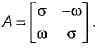The eigen values of A are

Detailed Solution for Test: State Variable Analysis- 2 - Question 1

Eigen values of A are given by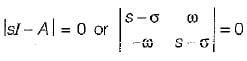or, (s - σ)2 + ω2 = 0
or,  s = σ ± jω
or,  s = σ + jω and s = σ - jω

Test: State Variable Analysis- 2 - Question 2

### The system equations are given by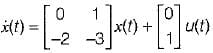y(t) = [1   0]x(t) The transfer function of the above system is

Detailed Solution for Test: State Variable Analysis- 2 - Question 2

Given,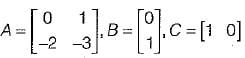Transfer function of the given system is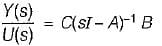Now,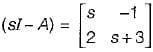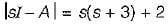= s2 + 3s + 2
Now,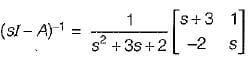Now,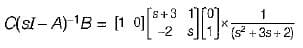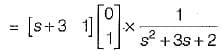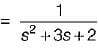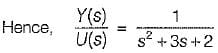Test: State Variable Analysis- 2 - Question 3

### Consider the following statements related to state space analysis of control systems: 1. The zeros of the system can be obtained from eigen value of the system matrix. 2. A system is said to be observable if every state x0 can be exactly determined from the measurement of the output ‘y’ over a finite interval of time 0 ≤ t ≤ tf. 3. The process by which transfer function changes to state diagram or state equations is called decomposition of the transfer function. 4. The state space techniques can be applied to linear and time invariant systems only. Which of the above statements are correct?

Detailed Solution for Test: State Variable Analysis- 2 - Question 3

The poles of the system can be obtained from eigen values of the system matrix. Hence, statement-1 is false.
State space techniques can be applied to linear or non-linear, time variant or time invariant systems.
Hence, statement-4 is false.

Test: State Variable Analysis- 2 - Question 4

Consider the system shown in figure below: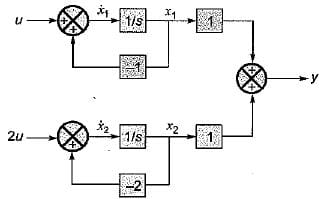The system is

Detailed Solution for Test: State Variable Analysis- 2 - Question 4

From given block diagram, the state equations can be written as:
x1 = -x1 + u and x2 = -2x2 + 2u
in matrix form,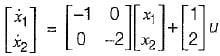Also, y = x1 + x2
In matrix form,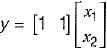Thus,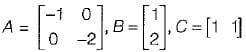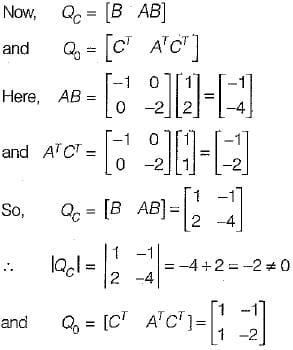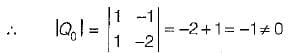Since, |Qc| ≠ 0 and |Q0| ≠ 0, therefore given system is both controllable and observable.

Test: State Variable Analysis- 2 - Question 5

Consider the following matrix: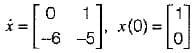x(t) is given by

Detailed Solution for Test: State Variable Analysis- 2 - Question 5

Given,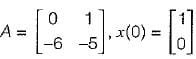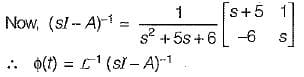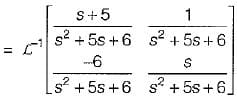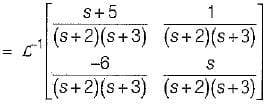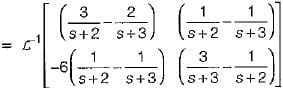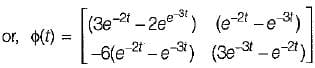So, x(t) = ϕ(t).x(0)
= state transition equation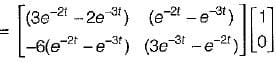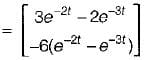Test: State Variable Analysis- 2 - Question 6

The transfer function of the system shown below is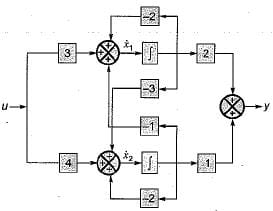Detailed Solution for Test: State Variable Analysis- 2 - Question 6

From given block diagram, the state equations can be written as: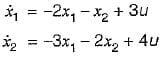Also, output equation is
y = 2x1 + x2
In matrix form, we have: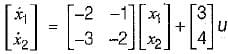and y = [2  1]x(t)
Now,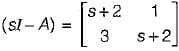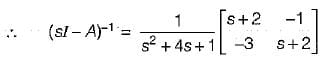∴ Transfer function,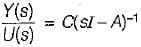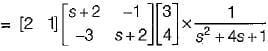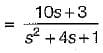Test: State Variable Analysis- 2 - Question 7

The state equation for the circuit shown below is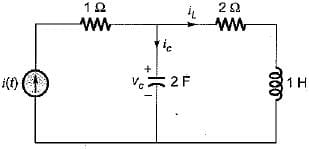Detailed Solution for Test: State Variable Analysis- 2 - Question 7

Let us select the state variables as Vc and iL.
Applying KVL in the mesh-2, we have: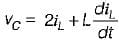or,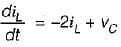.............(1)
Also, by applying KCL at the given node, we get: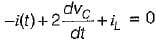or,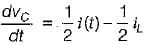..........(2)
From equations (1) and (2), state equations in matrix form can be written as: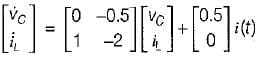Test: State Variable Analysis- 2 - Question 8

The state space representation of the system represented by the SFG shown below is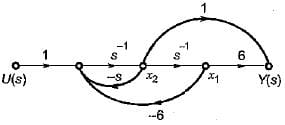Detailed Solution for Test: State Variable Analysis- 2 - Question 8

The state equations from the given signal flow graph can be written as: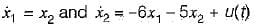In matrix form,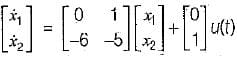Also, output is
y(t) = 6x1 + x2
In matrix form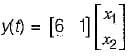Test: State Variable Analysis- 2 - Question 9

The state variable representation of a system is given by: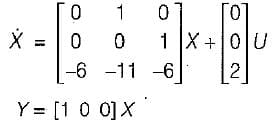The system is

Detailed Solution for Test: State Variable Analysis- 2 - Question 9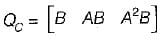Here,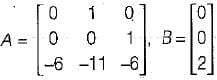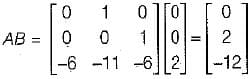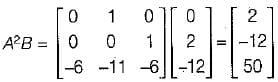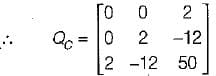So, IQCI = 0 - 0 + 2(0 - 4)
= - 8 ≠ 0
Hence, the system is controllable.
Also, Q0 = [CT ATCT (AT)2 CT]
Here,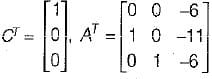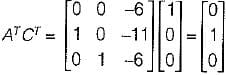and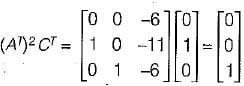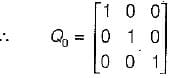So,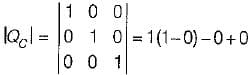= 1 ≠ 0
Since |Q0| ≠ 0, therefore given system is observable.

Test: State Variable Analysis- 2 - Question 10

The zeros of following system are located at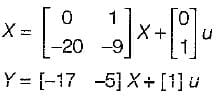Detailed Solution for Test: State Variable Analysis- 2 - Question 10

T.F.,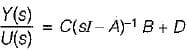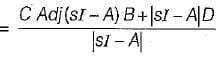Hence, zeros are at: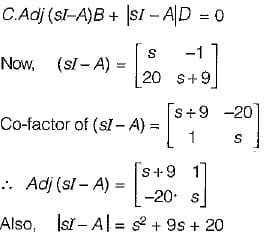So, zeros are at: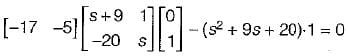or,  -17 -5s + s2 + 9s + 20 = 0
or,  s2 + 4s + 3 = 0
or,  (s+1) (s+3) = 0
or,  s = -1,- 3

## GATE Electrical Engineering (EE) 2024 Mock Test Series

23 docs|285 tests
Information about Test: State Variable Analysis- 2 Page
In this test you can find the Exam questions for Test: State Variable Analysis- 2 solved & explained in the simplest way possible. Besides giving Questions and answers for Test: State Variable Analysis- 2, EduRev gives you an ample number of Online tests for practice

## GATE Electrical Engineering (EE) 2024 Mock Test Series

23 docs|285 tests